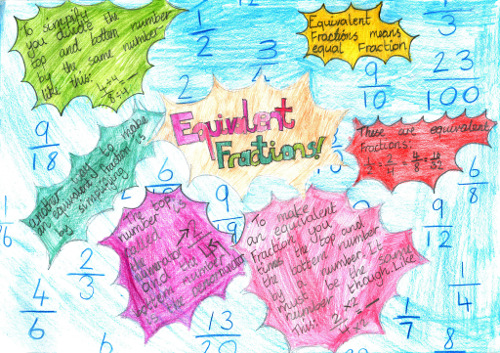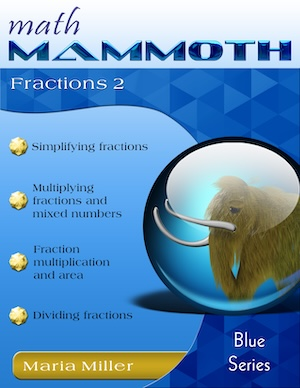^
You are here: HomeLessons →Why is fraction math so difficult

# Why are fractions so difficult for students?Photo by Steve Hodgson; licensed by CC BY-SA 2.0

As many teachers and parents know, learning the various fraction operations can be difficult for many children. It's not the concept of a fraction that is difficult — it is the various OPERATIONS with fractions: addition, subtraction, multiplication, division, comparing, simplifying, etc.

And the simple reason why learning the various fraction operations proves difficult for many students is the way they are typically taught, and how many there are. Just look at the amount of rules there are to learn about fractions!

 1. Fraction addition / subtraction — common denominators Add the numerators, and use the common denominator 2. Fraction addition / subtraction — different denominators First find a common denominator by taking the least common multiple of the denominators. Then convert all the addends to have this common denominator. Then add using the rule above. 3. Equivalent fractions Multiply both the numerator and denominator by a same number. 4. Convert a mixed number to a fraction Multiply the whole number part by the denominator and add the numerator to get the numerator. Use the common denominator as in the fractional part of the mixed number. 5. Convert an improper fraction to a mixed number Divide the numerator by the denominator to get the whole-number part. The remainder will be the numerator of the fractional part. The denominator is the same. 6. Simplify fractions Find the (greatest) common divisor of the numerator and denominator, and divide both by it. 7. Fraction multiplication Multiply the numerators and the denominators. 8. Fraction division Find the reciprocal of the divisor, and multiply by it. 9. Comparing fractions Convert the fractions so they have a common denominator. Then compare the numerators. 10. Convert fractions to decimals Divide using long division or a calculator.

If students simply try to memorize these rules without knowing where they came from, the rules will probably seem like a meaningless jungle. They probably won't seem to connect with anything about the operation, but instead are like "magic", or a random play where you multiply, divide, and do various things with the numerators and denominators to come up with the answer.

Students can then become blind followers of the rules, tossing numbers here and there — and getting answers of which they have no idea if they are reasonable or not. Besides, it is quite easy to forget these rules or remember them wrong — especially after 5-10 years.

## The solution: visual models

Instead of merely presenting a rule (as many books do), a better way is to use visual models or manipulatives. That way fractions become something real and concrete to the student, and not just a number on top of another without a meaning. The student will be able to estimate the answer before calculating, evaluate the reasonableness of the final answer, and perform many of the simplest operations mentally without knowingly applying any "rule."

Of course textbooks DO show visual models for fractions, and they DO show one or two examples of how a certain rule connects with a picture. But that is not enough! We need to have children solve lots of problems using either visual models or fraction manipulatives.

Another way is to ask them to DRAW fraction pictures for the problems. That way the students will form a mental visual model, and can think through the pictures.

For example, this video shows a visual method for equivalent fractions: that of splitting the pieces further into a certain number of new pieces:

If you think through pictures, you will easily see the need for multiplying or dividing both the numerator and denominator by the same number. But before voicing that rule, it is better that children get lots of 'hands-on' experiences with fraction pictures they draw themselves. They can have FUN splitting the pieces further (or conversely merging pieces together)! They may even find the rule THEMSELVES — and it will make sense. If they forget the rule later, they can always fall back to thinking about splitting the pieces and re-discover it.

Another example is the topic of adding unlike fractions (see video). The teacher can show how the pieces in the fractions need to be split further so that they are all the SAME KIND of pieces — and then we can add.

When first learning about this (say in 4th grade), there is no need to use the term "least common denominator". One can simply use pictures or manipulatives. Then in 5th grade, the students will add unlike fractions using manipulatives or by drawing pictures. After a while, some students might discover the rule about the common denominator, or in other words, what kind of pieces the fractions will need split into. In any case, they will certainly remember the rule better when they have been able to verify it themselves with numerous visual examples.

I'm not saying that the rules are not needed — because they are. You cannot get through algebra without knowing the actual rules for fraction operations. But by using visual models extensively in the beginning stages, the rules will make more sense, and if 10 years later you have forgotten the rules, you should still able to "do the math" with the pictures in you mind, and not consider fractions as something you just "cannot do".

## Do you need help with fractions?

First, check out my free videos for fraction operations:

Fraction videos, part 1
(mixed numbers, equivalent fractions, addition, subtraction, comparing)
Fraction videos, part 2
(simplifying, multiplying, and dividing fractions; fractions to decimals; ratios and fractions; a fractional part of a group of objects)

Secondly, these two worktexts will help you even further! Both of them explain fraction operations using a visual model of a pie, and let the students practice sufficiently with visual exercises so they will understand the concepts. Best meant for grade 5, when fraction operations are typically studied:Math Mammoth Fractions 1

A self-teaching worktext that covers fractions, mixed numbers, equivalent fractions, adding and subtracting like fractions and unlike fractions, adding and subtracting mixed numbers, comparing fractions, and finding a fractional part of a group.Math Mammoth Fractions 2

A self-teaching worktext, sequel to Math Mammoth Fractions 1. Covers simplifying fractions, multiplication and division of fractions and mixed numbers, converting fractions to decimals, and ratios and fractions.

I also sell a fractions worktext for grades 2-4, Introduction to Fractions, and a worktext for grade 6, Fractions & Decimals 3.

WAIT!

Receive my monthly collection of math tips & resources directly in your inbox — and get a FREE Math Mammoth book!You can unsubscribe at any time.

### Math Mammoth Tour

Confused about the different options? Take a virtual email tour around Math Mammoth! You'll receive:

An initial email to download your GIFT of over 400 free worksheets and sample pages from my books. Six other "TOURSTOP" emails that explain the important things and commonly asked questions concerning Math Mammoth curriculum. (Find out the differences between all these different-colored series!)

This way, you'll have time to digest the information over one or two weeks, plus an opportunity to ask me personally about the curriculum.
A monthly collection of math teaching tips & Math Mammoth updates (unsubscribe any time)### "Mini" Math Teaching Course

This is a little "virtual" 2-week course, where you will receive emails on important topics on teaching math, including:

- How to help a student who is behind
- Troubles with word problems
- Teaching multiplication tables
- Why fractions are so difficult
- The value of mistakes
- Should you use timed tests
- And more!

A GIFT of over 400 free worksheets and sample pages from my books right in the very beginning.A monthly collection of math teaching tips & Math Mammoth updates (unsubscribe any time)
Enter your email to receive math teaching tips, resources, Math Mammoth news & sales, humor, and more! I tend to send out these tips about once monthly, near the beginning of the month, but occasionally you may hear from me twice per month (and sometimes less often).• A GIFT of over 400 free worksheets and sample pages from my books.